Monday 08th August 2022CBSE Guess > Papers > Important Questions > Class XI > 2010 > Maths > Maths By Mr. Anil Kumar Tondak CBSE CLASS XI
Introduction to 3-D Geometry Q.1. Show that the points (-2, 3, 5), (1, 2, 3) and (7,0,-1) are collinear. Q.2. Find the equation of the set of points P, the sum of whose distances from A(4, 0, 0) and B (-4, 0,0) is equal to 10. Q.3. Find the equation of the set of points which are equidistant from the points (1, 2, 3) and (3, 2, –1). Q.4. Find the equation of set of points P such that PA2+ PB2 = 2k2 where A and B are the points (3, 4, 5) and (–1, 3, –7), respectively. Q.5. Find the coordinates of the point which divides the line segment joining the points (1, –2, 3) and (3, 4,–5) in the ratio 2 : 3 (i) internally, and (ii) externally. Q.6. Using section formula, prove that the three points (– 4, 6, 10), (2, 4, 6)and (14, 0, –2) are collinear. Q.7. Find the coordinates of the centroid of the triangle whose vertices are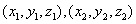and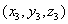. Q.8. Find the ratio in which the line segment joining the points (4, 8, 10) and (6, 10,-8) is divided by the YZ- plane. Q.9. Find the Coordinates of the points which trisect the line segment joining the points P (4, 2,-6) and Q(10, -16, 6). Q.10. Show that the points A(1, 2, 3), B(-1,-2,-1), C(2, 3, 2) and D(4, 7, 6) are the vertices of parallelogram ABCD, but it is not a rectangle. Q.11. If the origin is the centroid of the triangle PQR with vertices P (2a, 2, 6), Q (– 4, 3b, –10) and R(8, 14, 2c), then find the values of a, b and c. Q.12. Find the coordinates of a point on y-axis which are at a distance of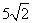from the point P(3, -2, 5). Q.13. A point R with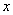-coordinate 4 lies on the line segment joining the points P(2, -3, 4) and Q(8, 0, 10). Find the coordinates of the point R. Q. 14. Find the equation of the circle with centre (-a, -b) and radius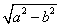Paper By Mr. Anil Kumar Tondak Email Id : [email protected] Ph No.: 9811363962